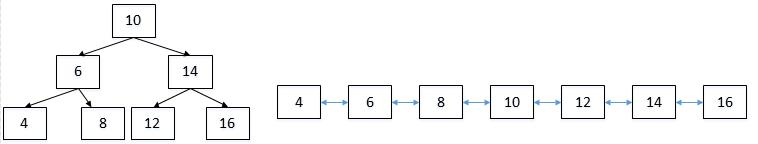## 题目6 二叉搜索树的后序遍历序列

### 代码

bool VerifySquenceOfBST(vector<int> sequence) { if(sequence.size()==0) return false; return IsTreeBST(sequence,0,sequence.size()-1);}bool IsTreeBST(vector<int> sequence, int start, int end){ if(end < start) return true; int i=start; for(;i<end;i++) { if(sequence[i]>sequence[end]) break; } for(int j=i;j<end;j++) { if(sequence[j]<sequence[end]) return false; } return IsTreeBST(sequence,start,i-1)&&IsTreeBST(sequence, i,end-1);}

## 题目7 二叉树中和为某一值的路径

### 题目解析

1访问结点8结点88
2访问结点5结点8，结点513
3访问结点3结点8，结点5，结点316
4回到结点5结点8，结点513
5访问结点9结点8，结点5，结点922
6返回结点5结点8，结点513
7回到结点8结点88
8访问结点14结点8，结点1422

vector<vector<int> > buffer

vector<int> tmp;

1. 加上当前结点的值，是否等于预设值了呢？（即(expectNumber-root->val)==0）；
2. 该结点是否是叶结点了呢？（root->left==NULL && root->right==NULL）

### 代码

vector<vector<int> > buffer;vector<int> tmp;vector<vector<int> > FindPath(TreeNode* root,int expectNumber) { if(root==NULL)//鲁棒性 return buffer; tmp.push_back(root->val); if((expectNumber-root->val)==0 && root->left==NULL && root->right==NULL) { buffer.push_back(tmp); } FindPath(root->left,expectNumber-root->val); FindPath(root->right,expectNumber-root->val); if(tmp.size()!=0) tmp.pop_back(); return buffer;}

## 题目8 复杂链表的复制

### 题目解析

void nodeClone(RandomListNode *head){ RandomListNode *pNode = head; while (pNode != NULL) { RandomListNode *pClone = new RandomListNode(pNode->label); pClone->next = pNode->next; pNode->next = pClone; pNode = pClone->next; }}

void connectRandom(RandomListNode *head){ RandomListNode *pNode = head; while (pNode != NULL) { RandomListNode *pClone = pNode->next; if (pNode->random) { pClone->random = pNode->random->next; } pNode = pClone->next; }}

RandomListNode *reconnect(RandomListNode *head){ RandomListNode *pNode = head; RandomListNode *result = head->next; while (pNode != NULL) { RandomListNode *pClone = pNode->next; pNode->next = pClone->next; pNode = pNode->next; if (pNode != NULL) pClone->next = pNode->next; } return result;}

## 题目9 二叉搜索树与双向链表

### 题目解析### 代码

TreeNode* Convert(TreeNode* pRootOfTree) { if (pRootOfTree == NULL)return NULL; TreeNode *pointer = NULL; convert2List(pRootOfTree, pointer); while (pointer->left!=NULL) { pointer = pointer->left; } return pointer; } void convert2List(TreeNode* pRoot,TreeNode *&pointer) { if (pRoot == NULL) { return; } { if (pRoot->left != NULL) { convert2List(pRoot->left,pointer); } pRoot->left = pointer; if (pointer != NULL) { pointer->right = pRoot; } pointer = pRoot; if (pRoot->right!=NULL) { convert2List(pRoot->right, pointer); } } }

## 题目10 字符串的排列

### 代码

vector<string> result;vector<string> Permutation(string str) { if(str.length()==0){ return result; } Permutation1(str,0); sort(result.begin(),result.end()); return result;}void Permutation1(string str,int begin){ if(begin == str.length()){ result.push_back(str); return ; } for(int i = begin; str[i]!='\0';i++){ if(i!=begin&&str[begin]==str[i]) continue; swap(str[begin],str[i]); Permutation1(str,begin+1); swap(str[begin],str[i]); } }